# Simulating Tensegrities

Simulation of tensegrities has two main parts. First, the general form of the tensegrity must be selected. This includes determining the number of struts, tendons, and how they connect. The second step, length calculation, is to determine lengths for the members of the tensegrity that will yield a static structure. Here I say lengths rather than the lengths because there are more than one unique set of lengths that will result in a stable structure.

## Connectivity Structure

Tensegrity structures come in many forms; there are endless configurations of struts and tendons that yield static structures. It is not trivial to devise a new tensegrity; in my experience doing so relies on past experience, looking at examples of tensegrities created by others, and attempting to generalize the patterns in existing tensegrities.

### Polyhedra-Induced Tensegrities

One way of coming up with tensegrity configurations is based on a derivation from polyhedra: the edges of the polyhedron are converted to struts, and adjacent struts are connected if the vertices of their parent edges were either the same, or adjacent vertices. Other variations on this idea exist and yield similar results with some aesthetic and structural differences.

The following video animates the procedure of turning a polyhedron (in the case of the video, an octahedron) into a tensegrity.

## Length Calculation

After deciding on the connectivity structure of the tensegrity, we must balance the lengths of the struts and tendons in order to yield a static structure. This can be thought of as holding the struts to fixed lengths and then shortening the tendons until they can no longer be shortened. Tensegrities constructed with elastic tendons literally perform this procedure, but for rigid tensegrities constructed with static tendons, the precise lengths must be calculated.

The procedure that I use to calculate tensegrity measurements is to model the tensegrity as a spring-mass system, with stiff springs in compression representing the struts, and weak springs in tension representing the tendons. Using elementary physics I model the system as an initial value problem with ordinary differential equations (ODEs). This technique has several advantages:

• The fixed-length struts are implicitly modeled and so no cumbersome algebraic constraints need to be introduced.
• The initial state of the system may be specified very loosely — we do not need to be close to the correct answer to find it.
• Numerical techniques for solving ODEs are widely known and studied in numerical analysis, and many solvers are available.

## Spring-Mass Systems

To model the springs I use Hooke’s Law.

### Basics

For a spring with resting lengthand stiffness constant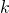, with one end fixed at the origin (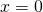) and the other (free) end at position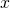, the spring exerts a force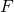of magnitudeWhen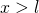, the spring is stretched, and the force is exerted inwards, and when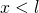, the spring is compressed, and the force is exerted outwards. The signed force is therefore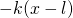.

Attaching a mass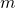to the free end of the spring and applying Newton’s second law, that the positionof the mass evolves according to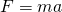, and putting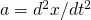, we have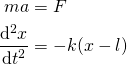### Free Masses

Next we model a system where both ends of the spring are free to move. Call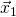the position (in two- or three-dimensional space) of one end and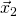the position of the other. Then the positions evolve according to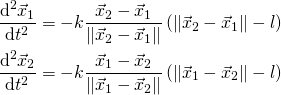### More Complicated

This pattern can be continued to model more complicated systems of springs. By introducing springs of different spring stiffnesses, we more strongly enforce that they tend towards their resting length. To model tensegrities I let the springs associated with struts have stiffness constant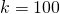, and springs associated with tendons have stiffness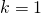.

## Relaxation

Through the above procedure, we obtain ODEs that model how the spring system will evolve in time given an initial configuration. To obtain a tensegrity, we wish to find a set of positions for the masses that result in a system where the masses do not move relative to one another. Rather than attempting to solve for a solution directly, I use a relaxation method where an initial guess is supplied, and the kinetics of the system evolve the configuration into a valid one.

### Friction

One method of bringing the system to rest is to add friction. This can be done in a number of ways, but typically the velocity is retarded in proportion to its magnitude. Adding friction will damp oscillations and lead the system to a resting state.

### Periodic Stopping

Another method of achieving a resting state is to periodically reset the velocity of all masses to zero. As the system evolves in time energy is converted between kinetic and potential (spring) energy. Periodically removing the kinetic energy brings the system to successively lower energy states, until eventually the system is at rest.